Home > A2C > Chapter 9 > Lesson 9.3.1 > Problem9-128

9-128.
1. Given the equation: 3x + yz = 6. Homework Help ✎

1. Draw a graph.

2. Is (1, 2, −1) on the graph? Justify your answer.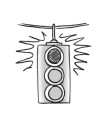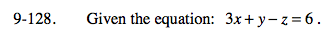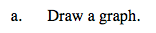Notice that there are three variables. Find the 3 intercepts.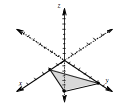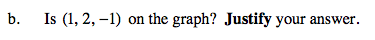Yes, it is a solution to the equation